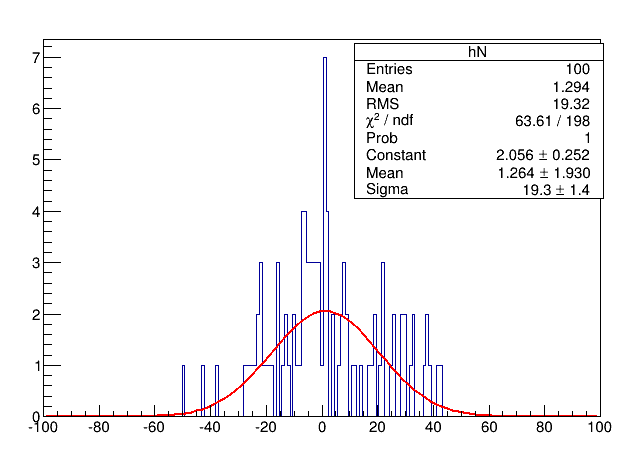# Chi2 calculation after likelihood fit

Hi,

I have a question regarding the Chi2 calculation after a likelihood fit.

I have a histogram with several empty bins that I fit using the standard Poisson likelihood (“L”) option. After the fit is performed, when I try and retrieve the Chi2 and NDF values for the fit I noticed some strange behaviour. (I am aware that the Chi2 test is not valid for empty or low-occupancy bins, but I am just trying to understand how it is calculated in ROOT)

The NDF value seems to include all bins in the fit range, whether they are empty or not.
The Chi2 value also seems to include all bins in the fit range whether they are empty or not, by assigning an error of 1 to all empty bins.

Is this an accurate description of the Chi2 and NDF calculation in ROOT after a likelihood fit ?
Is this the desired behavior ? If so, could someone point me to an explanation for the use of 1 as the error on an empty bin ?

Thank you

1 Like

In the chi2 method, empty bins should be skipped (not considered in the fit) unless you use the “WW” fit option.
In the likelihood method (the “L” or “WL” fit option), empty bins should always be treated “correctly”.

The issue I am having is that the default ROOT chi2 and NDF calculation after a likelihood fit does NOT skip empty bins.

To illustrate this issue I am attaching some code (run using 5.34/05):

TH1F* hN=new TH1F(“hN”,"",201,-100,100);
//Generates random distribution
int N=100;
TRandom3 *rand=new TRandom3();
for(int i=0;i<N;i++)
{
double x=rand->Gaus(2,20);
hN->Fill(x);
}

// Fit distribution using likelihood method
TFitResultPtr r=hN->Fit(“gaus”,“EMRLS”,"",-100,100);

//Retrieve Chi2 related values
double chi2=r->Chi2();
double ndf=r->Ndf();
double pvalue = r->Prob();
cout <<“This is ROOT: Chi2 " <<chi2<<”/"<<ndf<<endl;
cout <<"THis is ROOT: p-value "<<pvalue<<endl;

As you can see from the attached picture as well as the output to terminal, the NDF includes all bins, including empty bins in the range (corresponding to the NDF for the likelihood fit done).
The value of the Chi2 is also calculated by assuming an error of 1 for each empty bin - I have checked this with an independent calculation of my own.

This incorrect calculation of the Chi2 and NDF lead to an incorrect calculation of the p-value for the fit.

Again, I understand that the Chi2 statistic is not valid for data with empty bins, but I think the calculation in ROOT should at least be consistent (skip all empty bins for both Chi2 and NDF when calculating the p-value)1 Like